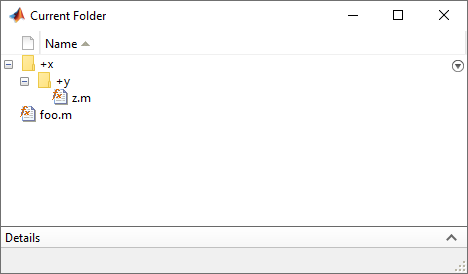## 生成的代码和 MATLAB 代码之间的差异

### 写入 `ans` 变量

```function foo %#codegen ans = 1; 2; disp(ans); end```

`foo`
`2`

`foo` 生成一个 MEX 函数。

`codegen foo`

`foo_mex`
`1`

### 逻辑短路

```function foo if [returnsFalse() & hasSideEffects()] end end function out = returnsFalse out = false; end function out = hasSideEffects out = true; disp('This is my string'); end```

`&` 运算符的第一个参数始终是 `false`，并确定条件表达式的值。因此，在 MATLAB 执行中，使用短路，并且不计算第二个参数。因此，`foo` 在执行期间不会调用 `hasSideEffects` 函数，也不会在命令行显示任何内容。

`foo` 生成一个 MEX 函数。调用生成的 MEX 函数 `foo_mex`

`foo_mex`
`This is my string`

### 循环索引溢出

• 循环索引递增 1。

• 结束值等于整数类型的最大值。

```N=intmax('int16') for k=N-10:N```

`for k=1:10`

• 循环索引递减 1。

• 结束值等于整数类型的最小值。

```N=intmin('int32') for k=N+10:-1:N```

`for k=10:-1:1`

• 循环索引递增或递减 1。

• 起始值等于整数类型的最小值或最大值。

• 结束值等于整数类型的最大值或最小值。

```M= intmin('int16'); N= intmax('int16'); for k=M:N % Loop body end```

```M= intmin('int16'); N= intmax('int16'); for k=int32(M):int32(N) % Loop body end```

• 循环索引按不等于 1 的值递增或递减。

• 在最后一次循环迭代中，循环索引不等于结束值。

### 使用单精度操作数对 `for` 循环进行索引

```function j = singlePIndex n = flintmax('single') + 2; j = single(0); for i = single(1):single(n) j = i; end end```

### 未执行的 `for` 循环的索引

• 如果在运行时提供 `for` 循环的开始和结束变量，则索引变量的值等于范围的开始值。以如下 MATLAB 代码为例：

```function out = indexTest(a,b) for i = a:b end out = i; end```

假设 `a``b` 作为 `1``-1` 传递。则 `for` 循环不会执行。在 MATLAB 中，`out` 被赋值为 [ ]。在生成代码中，`out` 被赋予 `a` 的值，即 `1`

• 如果在编译时间之前提供 `for` 循环的开始和结束值，则索引变量的值等于 `0`。以如下 MATLAB 代码为例：

```function out = indexTest for i = 1:-1 end out = i; end```

假设您调用此函数。在 MATLAB 中，`out` 被赋值为 [ ]。在生成代码中，`out` 被赋值为 `0`

### 字符大小

MATLAB 支持 16 位字符，但生成的代码以 8 位字符表示，这是适用于大多数嵌入式语言（如 C）的标准大小。请参阅代码生成中的字符编码

### 表达式中的计算顺序

• 修改持久性或全局变量的表达式

• 将数据显示到屏幕的表达式

• 将数据写入文件的表达式

• 修改句柄类对象属性的表达式

• 重写

`A = f1() + f2();`

```A = f1(); A = A + f2();```

使生成的代码在调用 `f2` 之前调用 `f1`

• 将多输出函数调用的输出赋给不相互依赖的变量。例如，重写

`[y, y.f, y.g] = foo;`

```[y, a, b] = foo; y.f = a; y.g = b; ```

• 当您访问元胞数组的多个元胞的内容时，将结果赋给不相互依赖的变量。例如，重写

```[y, y.f, y.g] = z{:}; ```

```[y, a, b] = z{:}; y.f = a; y.g = b; ```

### 构造函数句柄时的名称解析

MATLAB 和代码生成遵循不同优先级规则来解析符号 `@` 后面的名称。这些规则不适用于匿名函数。下表总结了这些优先级规则。

ExpressionMATLAB 中的优先顺序代码生成中的优先顺序

• MATLAB 生成错误。

• 生成代码将 `@x` 解释为 `x` 本身的函数句柄。```function out = foo x.y.z = @()'x.y.z is an anonymous function'; out = g(x); end function out = g(x) f = @x.y.z; out = f(); end```

```function out = z out = 'x.y.z is a package function'; end```

`foo` 生成一个 MEX 函数。分别调用生成的 MEX 函数 `foo_mex` 和 MATLAB 函数 `foo`

```codegen foo foo_mex foo```
```ans = 'x.y.z is an anonymous function' ans = 'x.y.z is a package function'```

### 删除数组元素所生成的空数组的大小

```coder.varsize('X',[4,4],[1,1]); X = zeros(2); X(:) = []; ```
`0-by-0``1-by-0`

```coder.varsize('X',[1,4],[0,1]); X = zeros(1,4); X(:) = [];```
`0-by-0``1-by-0`

```coder.varsize('X',[4,1],[1,0]); X = zeros(4,1); X(:) = [];```
`0-by-0``0-by-1`

```coder.varsize('X',[4,1],[1,0]); X = zeros(4,1); for i = 1:4 X(1)= []; end```
`1-by-0``0-by-1`

### 单精度和双精度操作数的二元按元素运算

```function out = foo(a,b) out = a + b; end```

```s1 = single(1.4e32); d1 = -5.305e+32; codegen foo -args {s1, d1} ```

```ml = foo(s1,d1); mlc = foo_mex(s1,d1); ml == mlc```
```ans = logical 0```

### NaN 和无穷

`NaN``Inf` 值在数学上没有意义时，它们在生成的代码中的模式可能不会与在 MATLAB 代码中完全相同。例如，如果 MATLAB 输出包含 `NaN`，生成的代码的输出也应包含 `NaN`，但不一定在相同的位置。

`NaN` 的位模式在 MATLAB 代码输出和生成的代码输出之间可能有所不同，这是因为用于生成代码的 C99 标准数学库未对所有实现中的 `NaN` 指定唯一位模式。请避免比较不同实现中的位模式，例如，在 MATLAB 输出和 SIL 或 PIL 输出之间进行比较。

### 代码生成目标

`coder.target` 函数在 MATLAB 中返回的值与在生成的代码中返回的值不同。这是为了帮助您确定您的函数是在 MATLAB 中执行，还是已针对仿真或代码生成目标进行编译。请参阅 `coder.target`

### 具有 set 方法的嵌套属性赋值中的 MATLAB 类

```x.pa.pb.pc = 0; ```

### MATLAB 句柄类析构函数

• 有些独立对象在 MATLAB 中的销毁顺序可能与生成的代码中的顺序不同。

• 生成的代码中的对象的生命周期可能与它们在 MATLAB 中的生命周期不同。

• 生成的代码不销毁部分构造的对象。如果句柄对象在运行时未完全构造，则生成的代码将生成错误消息，但不为该对象调用 `delete` 方法。对于 System object™，如果在 `setupImpl` 中存在运行时错误，则生成的代码不为该对象调用 `releaseImpl`

MATLAB 确实会调用 `delete` 方法来销毁部分构造的对象。

### 将使用连续一元运算符的字符串转换为 `double`

```function out = foo(op) out = double(op + 1); end```

## 支持